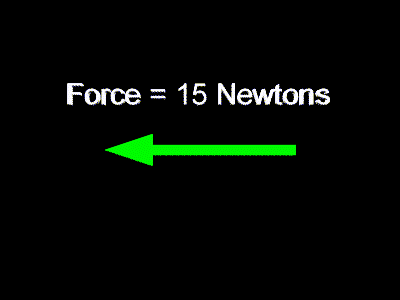# Forces in General

A force is a push or a pull.

When we say that there is a force on an object we mean that there is a push or a pull on the object. Some outside agent is placing this push or pull on the object.

The metric unit for force is a Newton, English is pound.

In the metric system forces are measured in units of Newtons. This unit is named after Isaac Newton.

In the English system forces are measured in units of pounds.

A Newton of force is much smaller than a pound of force. It takes about 4.5 Newtons to equal a force of one pound. So, if you usually state your weight in pounds, then you could figure your weight in the Newtons by multiplying those pounds by 4.5.

Forces are vectors.

Forces are vectors. That is, they are quantities that have both size and direction. To completely describe a force you need to state its size, or strength, and also state its direction.

Forces can be symbolized with arrows, as can all vectors. The arrow in the picture below is meant to show a force with the size of 15 Newtons directed toward the left.One must understand vectors to understand forces.

Custom Search